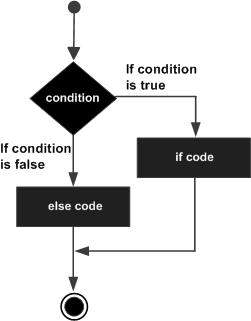# Elixir - If else statement

An if..else statement consists of a Boolean expression followed by one or more statements. This is further followed by an else statement with one or more statements.

## Syntax

The syntax of an if..else statement is as follows −

```if boolean-statement do
#Code to be executed if condition is satisfied
else
#Code to be executed if condition is not satisfied
end
```

If the Boolean expression evaluates to true, then the block of code inside the if statement will be executed. If Boolean expression evaluates to false, then the code after the else keyword of the given if statement will be executed.

## Flow Diagram## Example

```a = false
if a === true do
IO.puts "Variable a is true!"
else
IO.puts "Variable a is false!"
end
IO.puts "Outside the if statement"
```

The above program will generate the following result.

```Variable a is false!
Outside the if statement
```
elixir_decision_making.htm